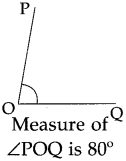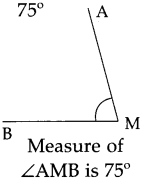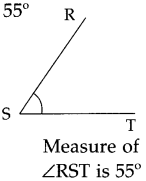# Maharashtra Board Class 5 Maths Solutions Chapter 6 Angles Problem Set 26

Balbharti Maharashtra Board Class 5 Maths Solutions Chapter 6 Angles Problem Set 26 Textbook Exercise Important Questions and Answers.

## Maharashtra State Board Class 5 Maths Solutions Chapter 6 Angles Problem Set 26

Question 1.
Draw and name the following angles with the help of a protractor.

(1) 60°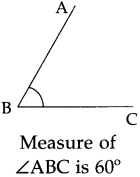(2) 120°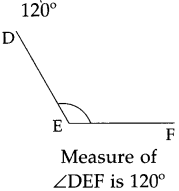(3) 90°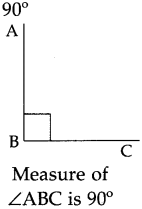(4) 150°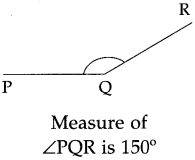(5) 30°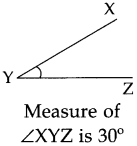(6) 165°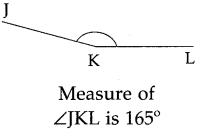(7) 45°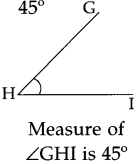Types of angles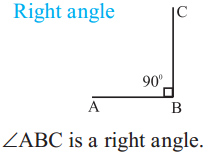∠ABC is a right angle.
Angles of measure 90° are called right angles.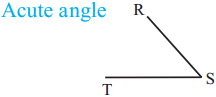∠RST measures less than 90°, that is, less than a right angle.
An angle which measures less than a right angle is called an acute angle.
∠RST is an acute angle.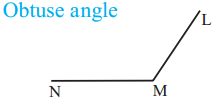∠LMN measures more than 90°, that is, more than a right angle.
An angle which measures more than a right angle is called an obtuse angle.
∠LMN is an obtuse angle.

Activity :

Making a right angle by folding
(1) Fold a sheet of paper roughly in half.
(2) Make another fold in the paper at any point on the first fold, as shown in the picture.
(3) Now unfold the paper. You will find two lines. The angle between those two lines will be a right angle.

With the help of a protractor, verify that the measure of this angle is 90°.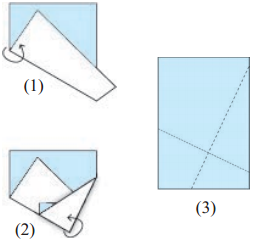Parallel and perpendicular lines
Parallel linesThe bars on the window in the picture are parallel to each other.
The steps on the ladder in the picture are parallel to each other.
The vertical legs of the ladder are parallel to each other.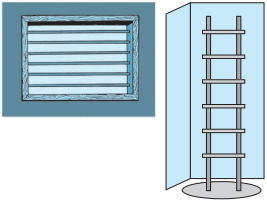1. Take a rectangular piece of paper.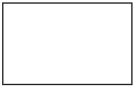2. Fold it in such a way that one edge falls exactly on the opposite edge.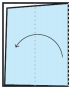3. Make another fold in the same way.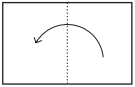4. Unfold the paper and trace the lines made by the folds, with a pencil.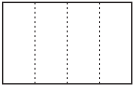___________ The lines traced with the pencil are parallel to each other.
______________________ The lines shown alongside are not of equal length, yet they are parallel to each other.

Parallel lines do not intersect, that is, they do not cut each other, no matter how far they are extended on either side.

Take a ruler as shown in the picture.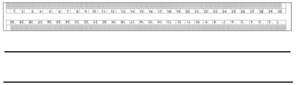Using a pencil, draw lines along both sides of the ruler. Put the ruler aside. The two lines are parallel to each other.
In this way, we can use several rectangular objects to draw parallel lines.Perpendicular lines

We have seen many objects standing straight on the ground. These objects form a right angle with their shadows.

For example, the angle formed by a pole and its shadow on the ground is 90° or a right angle. Similarly, adjacent sides of wooden planks or books also form angles of 90°.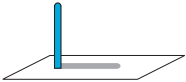When two lines form an angle of 90° with each other, they are said to be perpendicular to each other. To show that two lines are perpendicular, a symbol as shown the figure is drawn between them.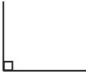Measure the angle between any two adjacent sides of your notebook. Since it is a right angle, the two sides are perpendicular to each other.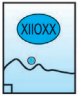Look at this picture of a page of a notebook.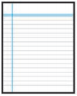The horizontal lines on the paper are parallel to each other. However, the vertical margin line on the side forms a right angle with the horizontal lines, therefore, it is perpendicular to the horizontal lines.